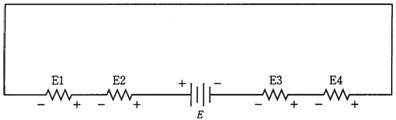## Kirchhoff’s second law Assignment Help

Assignment Help: >> Electrical Engineering - Kirchhoff’s second law

Kirchhoff's second law:

The sum of all the voltages, from some fixed point and return there from the opposite direction as you go round the circuit, and taking polarity into account, is always zero.

This might sound strange to you, there is voltage in your electric hair dryer/radio/ computer. Yes, there is, between different points. But no point can have an EMF w r t itself. This is so simple that it is laughable. A point in a circuit is shorted out to itself.

What Kirchhoff was saying, when he wrote his 2nd law, is a more general version of the 2nd and 3rd points mentioned previously. The reason he gave was that the voltage cannot appear out of anywhere, nor can it vanish. All the potential differences should balance out in any circuit, does not matter how complex and does not matter how many branches there are.

The Kirchhoff's Second Law is an alternative name might be the law of conservation of voltage.

Consider the rule you have learned about the series circuits already: The voltages across all individual resistors add up to the supply voltage. Yes, but the polarities of the EMFs across the resistors are opposite to the battery. It is a uncertain thing, but it becomes clear when a series circuit is drawn with all the components, including the battery or other EMF source, in line with each other, as in the given figure.

Problem :1

Suppose the 4 resistors have values of 50, 60, 70 and 80Ω, and that the current through them is 500 mA. What is the supply voltage, E?

Find the voltages E1, E2, E3, and E4 across each of the resistors. This is done via Ohm's Law. In the case of E1, say with the 50-Ω resistor, calculate E1 = 0. 500*50 = 25V. In the same way, you can calculate E2 = 30V, E3 = 35V, and E4 = 40V. The supply voltage is the sum E1+E2+E3+E4=25+30+35+40 V = 130 V.

Kirchhoff's Second Law tells us that the polarities of the voltages across the resistors are in the opposite direction from that of the supply in the above example.Figure--   Kirchhoff's Second Law. The sum of voltages across the resistors is equal to, but has opposite polarity from, the supply voltage E. Therefore E=E1+E2+E3+E4 = 0.